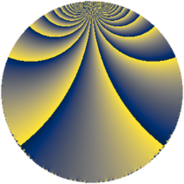# Properties

 Label 648.4.qLevel $648$ Weight $4$ Character orbit 648.q Rep. character $\chi_{648}(73,\cdot)$ Character field $\Q(\zeta_{9})$ Dimension $162$ Sturm bound $432$

# Related objects

## Defining parameters

 Level: $$N$$ $$=$$ $$648 = 2^{3} \cdot 3^{4}$$ Weight: $$k$$ $$=$$ $$4$$ Character orbit: $$[\chi]$$ $$=$$ 648.q (of order $$9$$ and degree $$6$$) Character conductor: $$\operatorname{cond}(\chi)$$ $$=$$ $$27$$ Character field: $$\Q(\zeta_{9})$$ Sturm bound: $$432$$

## Dimensions

The following table gives the dimensions of various subspaces of $$M_{4}(648, [\chi])$$.

Total New Old
Modular forms 2016 162 1854
Cusp forms 1872 162 1710
Eisenstein series 144 0 144

## Trace form

 $$162q + O(q^{10})$$ $$162q - 75q^{11} + 204q^{17} - 156q^{23} + 126q^{29} - 1260q^{35} + 1191q^{41} + 513q^{43} + 1350q^{47} - 594q^{49} - 1908q^{53} - 966q^{59} + 54q^{61} + 1800q^{65} + 1161q^{67} - 3084q^{77} + 1410q^{83} + 1089q^{89} - 3522q^{95} - 81q^{97} + O(q^{100})$$

## Decomposition of $$S_{4}^{\mathrm{new}}(648, [\chi])$$ into newform subspaces

The newforms in this space have not yet been added to the LMFDB.

## Decomposition of $$S_{4}^{\mathrm{old}}(648, [\chi])$$ into lower level spaces

$$S_{4}^{\mathrm{old}}(648, [\chi]) \cong$$ $$S_{4}^{\mathrm{new}}(27, [\chi])$$$$^{\oplus 8}$$$$\oplus$$$$S_{4}^{\mathrm{new}}(54, [\chi])$$$$^{\oplus 6}$$$$\oplus$$$$S_{4}^{\mathrm{new}}(81, [\chi])$$$$^{\oplus 4}$$$$\oplus$$$$S_{4}^{\mathrm{new}}(108, [\chi])$$$$^{\oplus 4}$$$$\oplus$$$$S_{4}^{\mathrm{new}}(162, [\chi])$$$$^{\oplus 3}$$$$\oplus$$$$S_{4}^{\mathrm{new}}(216, [\chi])$$$$^{\oplus 2}$$$$\oplus$$$$S_{4}^{\mathrm{new}}(324, [\chi])$$$$^{\oplus 2}$$